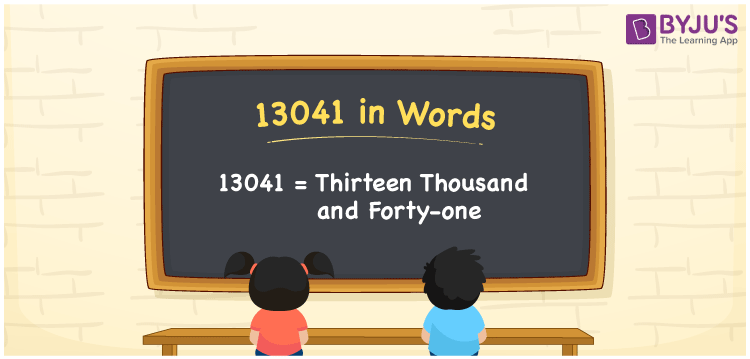# 13041 in Words

13041 in words is written as “Thirteen thousand and forty-one”. 13041 has five digits and its place values help to write the number name. Also, it is a cardinal number. For example, there are 13041 students in a school. Now, let us have a look at the procedure of writing the number 13041 in words in this article.

 13041 in Words: Thirteen Thousand and Forty-one. Thirteen Thousand and Forty-one in Numerical Form: 13041.

## 13041 in English Words## How to Write 13041 in Words?

The following place value table helps to write the number 13041 in words.

 Ten-thousands Thousands Hundreds Tens Ones 1 3 0 4 1

The expanded form of 13041 is as follows:

= 1 × Ten thousand + 3 × Thousand + 0 × Hundred + 4 × Ten + 1 × One

= 1 × 10000 + 3 × 1000 + 0 × 100 + 4 × 10 + 1 × 1

= 10000 + 3000 + 40 + 1

= 13041

= Thirteen thousand and forty-one

Hence, 13041 in words is thirteen thousand and forty-one.

13041 in words – Thirteen thousand and forty-one

Is 13041 an odd number? – Yes

Is 13041 an even number? – No

Is 13041 a perfect square number? – No

Is 13041 a perfect cube number? – No

Is 13041 a prime number? – No

Is 13041 a composite number? – Yes

## Frequently Asked Questions on 13041 in Words

Q1

### Write 13041 in words.

13041 in words is thirteen thousand and forty-one.

Q2

### Simplify 13000 + 41, and express it in words.

Simplifying 13000 + 41, we get 13041. Hence, 13041 in words is thirteen thousand and forty-one.

Q3

### Is 13041 a composite number?

Yes, 13041 is a composite number.